# Use Biot-Savart law to derive the expression for the magnetic field

Use Biot-Savart law to derive the expression for the magnetic field on the axis of a current carrying circular loop of radius R. Draw the magnetic field lines due to a circular wire carrying current.

Let us consider a circular loop of radius a with centre C. Let the plane of the coil be perpendicular to the plane of the paper and current I be flowing in the direction shown. Suppose P is any point on the axis at direction r from the centre.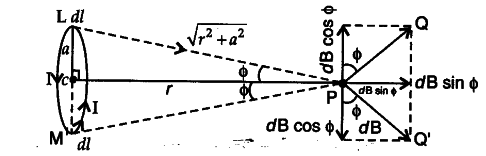Let us consider a current element dl on top (L) where, current comes out of paper normally whereas at bottom (M) enters into the plane paper normally.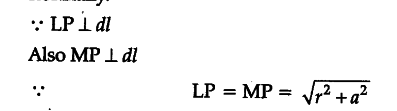Now, magnetic field at P due to current element at L according to Biot-Savart Law,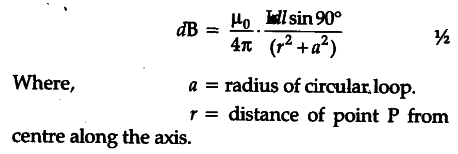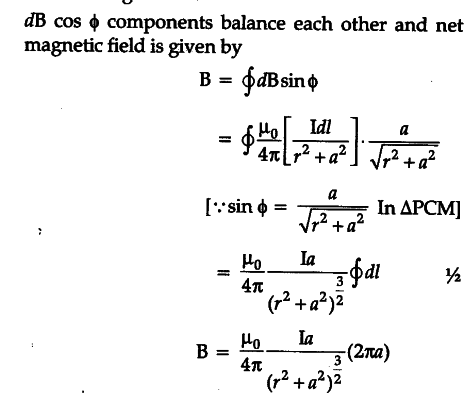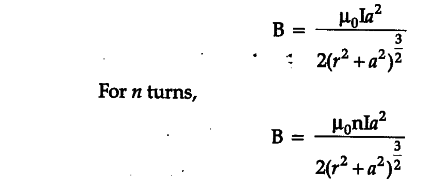Magnetic field due to Circular wire carrying current I.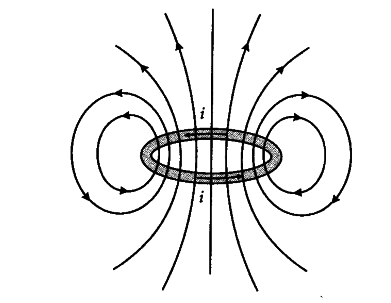1 Like